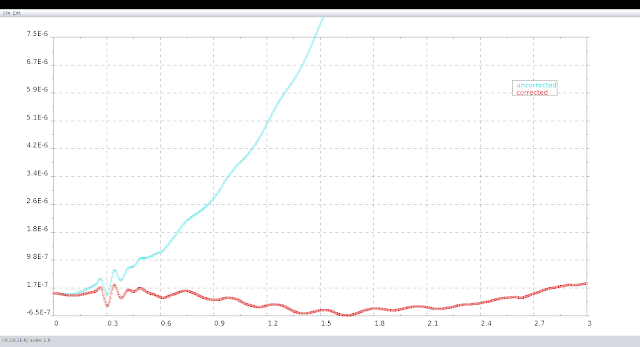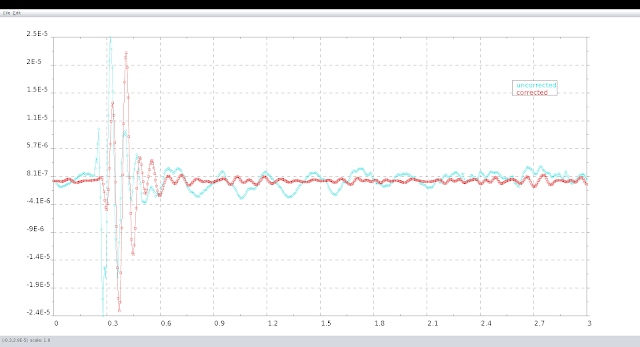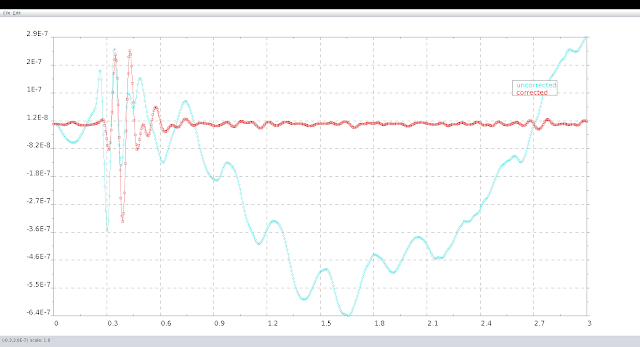### Baseline correction and filtering of acceleration record

In this post we see some processing of a recorded acceleration signal taken from some instrument placed on an old building of Rethymno at the island of Crete (Greece). We will successively use both baseline correction through the usual polynomial detrending and filtering. The acceleration original record can be seen in the plot of Fig. 1, entitled there as "uncorrected" even if it is hardly distinguished from the "corrected" one when only detrending is used as we see in what follows.

Baseline correction takes place using a polynomial detrending approach in order to correct the vibration acceleration signals with inconsistent initial velocity and displacement. We can choose the order of the polynomial to be used which for that example has been taken as a linear one (the variable, order=1, in the code). The Apache Common Maths fitting has been used (import of the necessary class is sufficient, we do not need to load any jar file since it is by default active in SDE).Figure 1: Acceleration record and its detrended signalFigure 2: Velocity after time integration of accelerationFigure 3: Displacement after double time integration of acceleration

Filtering is made possible loading the jar file of iirj an efficient IIR filter library written in JAVA. There is the option to use several filter types (Butterworth, Chebyshev, Bessel) and choosing the filter configuration (Lowpass Highpass, Bandpass, Bandstop). Here we have adopted the Butterworth type with a Bandpass of order equal to 4 and central frequency that of 12.55Hz with a width of 12.45Hz. To do so we need first (having the necessary library already loaded) to import the appropriate Butterworth class.
In the plots of Fig. 4 to 6 that follow, the uncorrected case is the one where only detrending has been applied while the corrected is the one of both detrending and filtering affect.Figure 4: Acceleration detrended record and its filtered signalFigure 5: Velocities after time integration of accelerations of Fig. 4Figure 6: Displacements after double time integration of accelerations of Fig. 4
The code of the above is embedded into the script file.